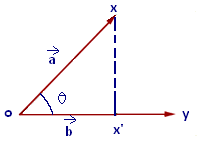# Vector Projection Formula

The vector projection is of two types: Scalar projection that tells about the magnitude of vector projection and the other is the Vector projection which says about itself and represents the unit vector.If the vector veca is projected on vecb then Vector Projection formula is given below:

$\large proj_{b}\,a=\frac{\vec{a}\cdot\vec{b}}{\left|\vec{b}\right|^{2}}\;\vec{b}$

The Scalar projection formula defines the length of given vector projection and is given below:

$\large proj_{b}\,a=\frac{\vec{a}\cdot\vec{b}}{\left|\vec{a}\right|}$

### Vector Projection Problems

Below are problems based on vector projection which may be helpful for you.

Question 1: Find the vector projection of

$$\begin{array}{l}5\,\vec{i}-4\,\vec{j}+\vec{k}\end{array}$$
along the vector
$$\begin{array}{l}3\,\vec{i}-2\,\vec{j}+4\,\vec{k}\end{array}$$
?

Solution:

Given:

$$\begin{array}{l}\vec{a}=5\,\vec{i}-4\,\vec{j}+\vec{k}\end{array}$$
$$\begin{array}{l}\vec{b}=3\,\vec{i}-2\,\vec{j}+4\,\vec{k}\end{array}$$

So, we have:

$$\begin{array}{l}\vec{a}\cdot\vec{b}=\left(a_{1}\,a_{2}+b_{1}\,b_{2}+c_{1}\,c_{2}\right)\end{array}$$
= (5(3) + (-4)(-2) + (1)(4))
= 15 + 8 + 4 = 27

$$\begin{array}{l}\left|\vec{b}\right|=\sqrt{3^{2}+\left(-2\right)^{2}+\left(4\right)^{2}}\end{array}$$

$$\begin{array}{l}=\sqrt{9+4+16}\end{array}$$

$$\begin{array}{l}=\sqrt{29}\;\left|\vec{b}\right|^{2}\end{array}$$
= 29.

The Vector projection is given by

$$\begin{array}{l}proj_{b}\,a= \frac{\vec{a}\cdot\vec{b}}{\left|\vec{b}\right|^{2}}\vec{b}\end{array}$$
$$\begin{array}{l}=\frac{9}{29}\left(3,-2,4\right)\end{array}$$
$$\begin{array}{l}=\frac{1}{29}\left(27,-18,36\right)\end{array}$$

The Projection of a on b are:

$$\begin{array}{l}\left(\frac{27}{29},\frac{-18}{29},\frac{36}{29}\right)\end{array}$$